# A note on LSTM Networks

## Recurrent Neural Networks

Humans don’t start their thinking from scratch every second. As we read this essay, we understand each word based on our understanding of previous words. We don’t throw everything away and start thinking from scratch again. Our thoughts have persistence.

Traditional neural networks can’t do this, and it seems like a major shortcoming. For example, imagine you want to classify what kind of event is happening at every point in a movie. It’s unclear how a traditional neural network could use its reasoning about previous events in the film to inform later ones.

Recurrent neural networks address this issue. They are networks with loops in them, allowing information to persist.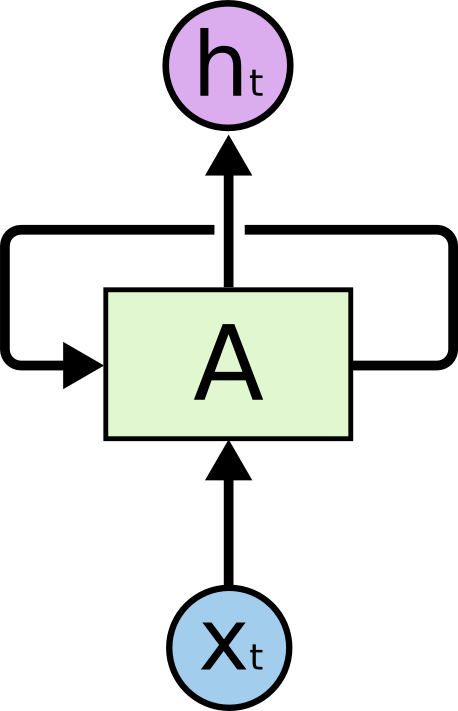Recurrent Neural Networks have loops.

In the above diagram, a chunk of neural network, *A*A, looks at some input *xt*xt and outputs a value *ht*ht. A loop allows information to be passed from one step of the network to the next.

These loops make recurrent neural networks seem kind of mysterious. However, if you think a bit more, it turns out that they aren’t all that different than a normal neural network. A recurrent neural network can be thought of as multiple copies of the same network, each passing a message to a successor. Consider what happens if we unroll the loop: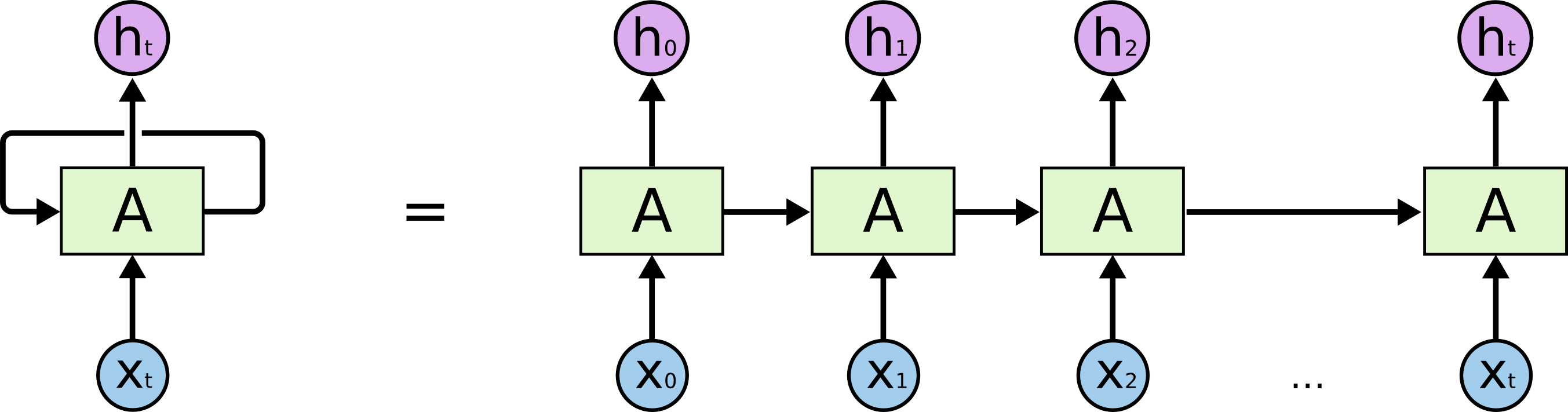An unrolled recurrent neural network.

This chain-like nature reveals that recurrent neural networks are intimately related to sequences and lists. They’re the natural architecture of neural network to use for such data.

An RNN works like this; First words get transformed into machine-readable vectors. Then the RNN processes the sequence of vectors one by one.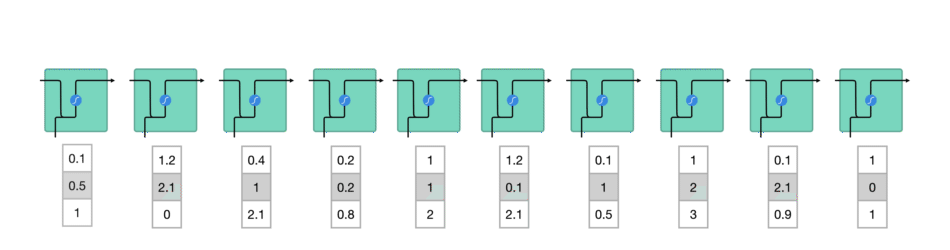Processing sequence one by one

While processing, it passes the previous hidden state to the next step of the sequence. The hidden state acts as the neural networks memory. It holds information on previous data the network has seen before.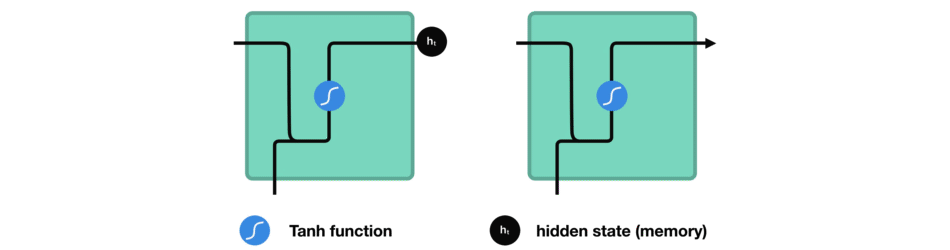Passing hidden state to next time step

Let’s look at a cell of the RNN to see how you would calculate the hidden state. First, the input and previous hidden state are combined to form a vector. That vector now has information on the current input and previous inputs. The vector goes through the tanh activation, and the output is the new hidden state, or the memory of the network.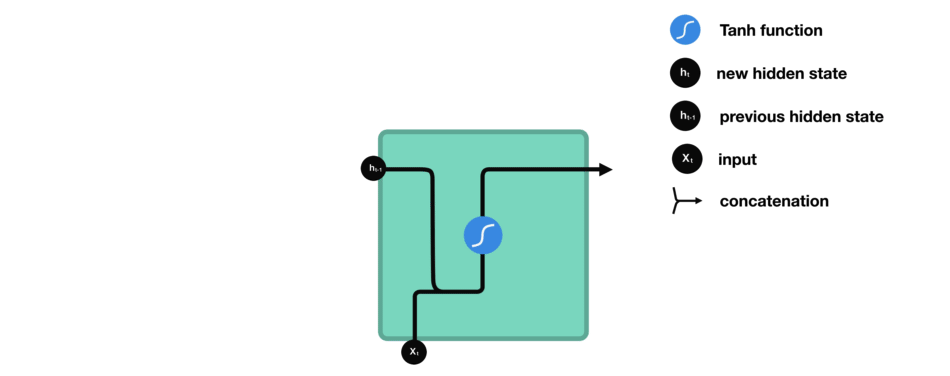RNN Cell

## Tanh activation

The tanh activation is used to help regulate the values flowing through the network. The tanh function squishes values to always be between -1 and 1.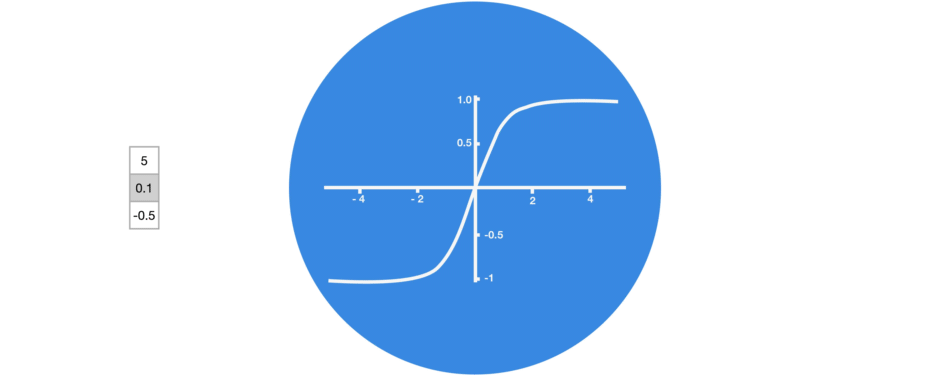Tanh squishes values to be between -1 and 1

When vectors are flowing through a neural network, it undergoes many transformations due to various math operations. So imagine a value that continues to be multiplied by let’s say 3. You can see how some values can explode and become astronomical, causing other values to seem insignificant.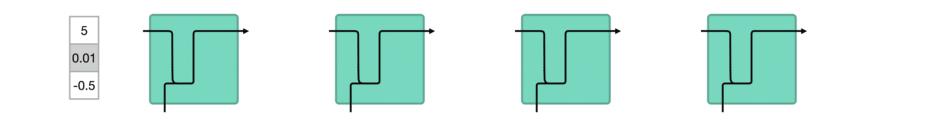vector transformations without tanh

A tanh function ensures that the values stay between -1 and 1, thus regulating the output of the neural network. You can see how the same values from above remain between the boundaries allowed by the tanh function.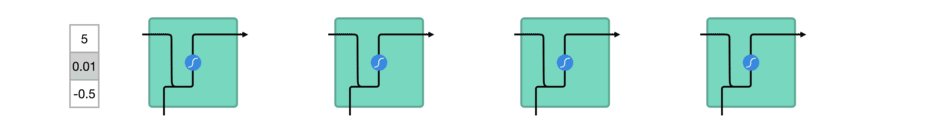vector transformations with tanh

So that’s an RNN. It has very few operations internally but works pretty well given the right circumstances (like short sequences).

# The Problem, Short-term Memory

Recurrent Neural Networks suffer from short-term memory. If a sequence is long enough, they’ll have a hard time carrying information from earlier time steps to later ones. So if you are trying to process a paragraph of text to do predictions, RNN’s may leave out important information from the beginning.

During back propagation, recurrent neural networks suffer from the vanishing gradient problem. Gradients are values used to update a neural networks weights. The vanishing gradient problem is when the gradient shrinks as it back propagates through time. If a gradient value becomes extremely small, it doesn’t contribute too much learning.

So in recurrent neural networks, layers that get a small gradient update stops learning. Those are usually the earlier layers. So because these layers don’t learn, RNN’s can forget what it seen in longer sequences, thus having a short-term memory.

# LSTM’s and GRU’s as a solution

LSTM ’s and GRU’s were created as the solution to short-term memory. They have internal mechanisms called gates that can regulate the flow of information.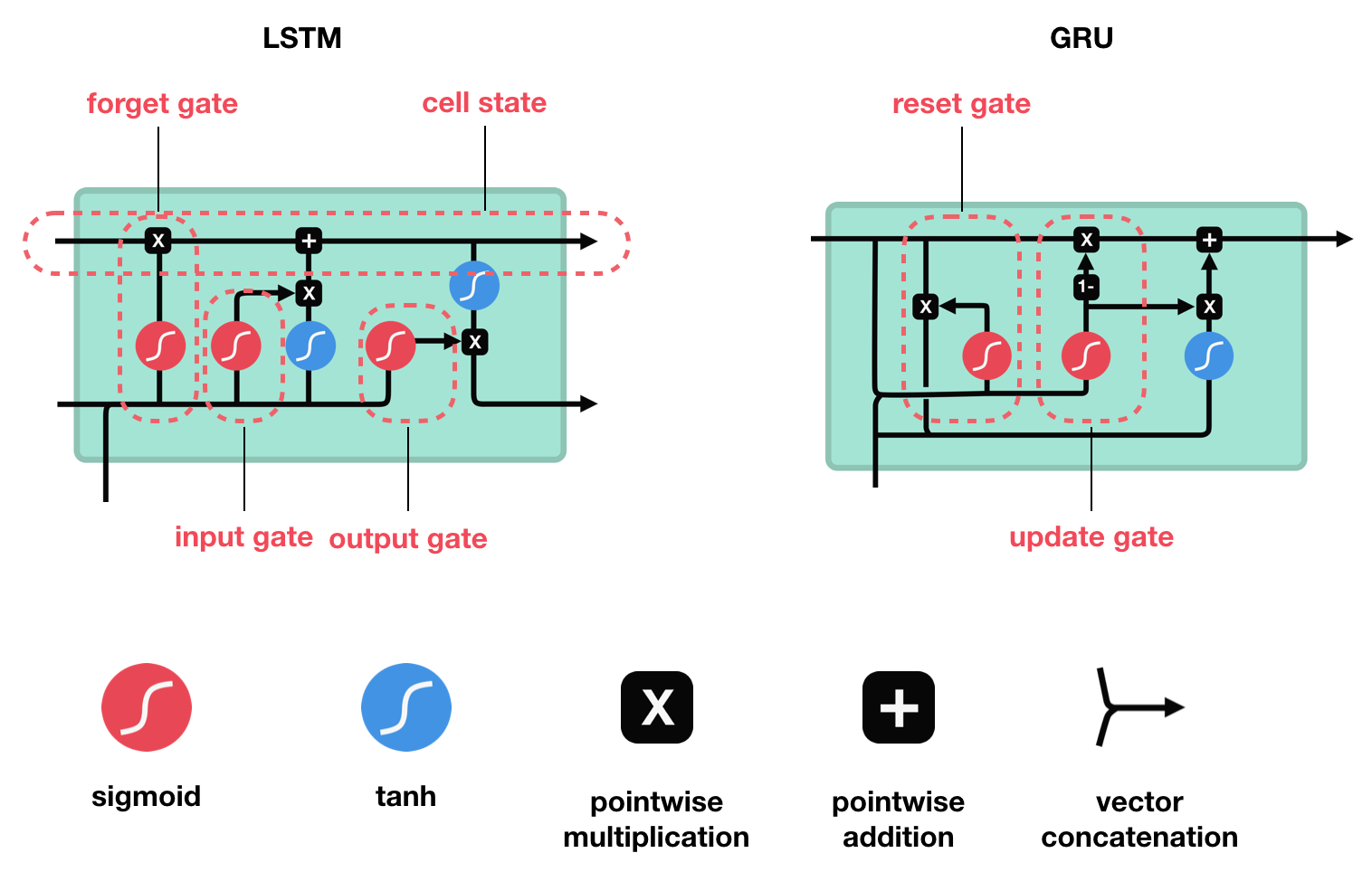These gates can learn which data in a sequence is important to keep or throw away. By doing that, it can pass relevant information down the long chain of sequences to make predictions. Almost all state of the art results based on recurrent neural networks are achieved with these two networks. LSTM’s and GRU’s can be found in speech recognition, speech synthesis, and text generation. You can even use them to generate captions for videos.

# LSTM

An LSTM has a similar control flow as a recurrent neural network. It processes data passing on information as it propagates forward. The differences are the operations within the LSTM’s cells.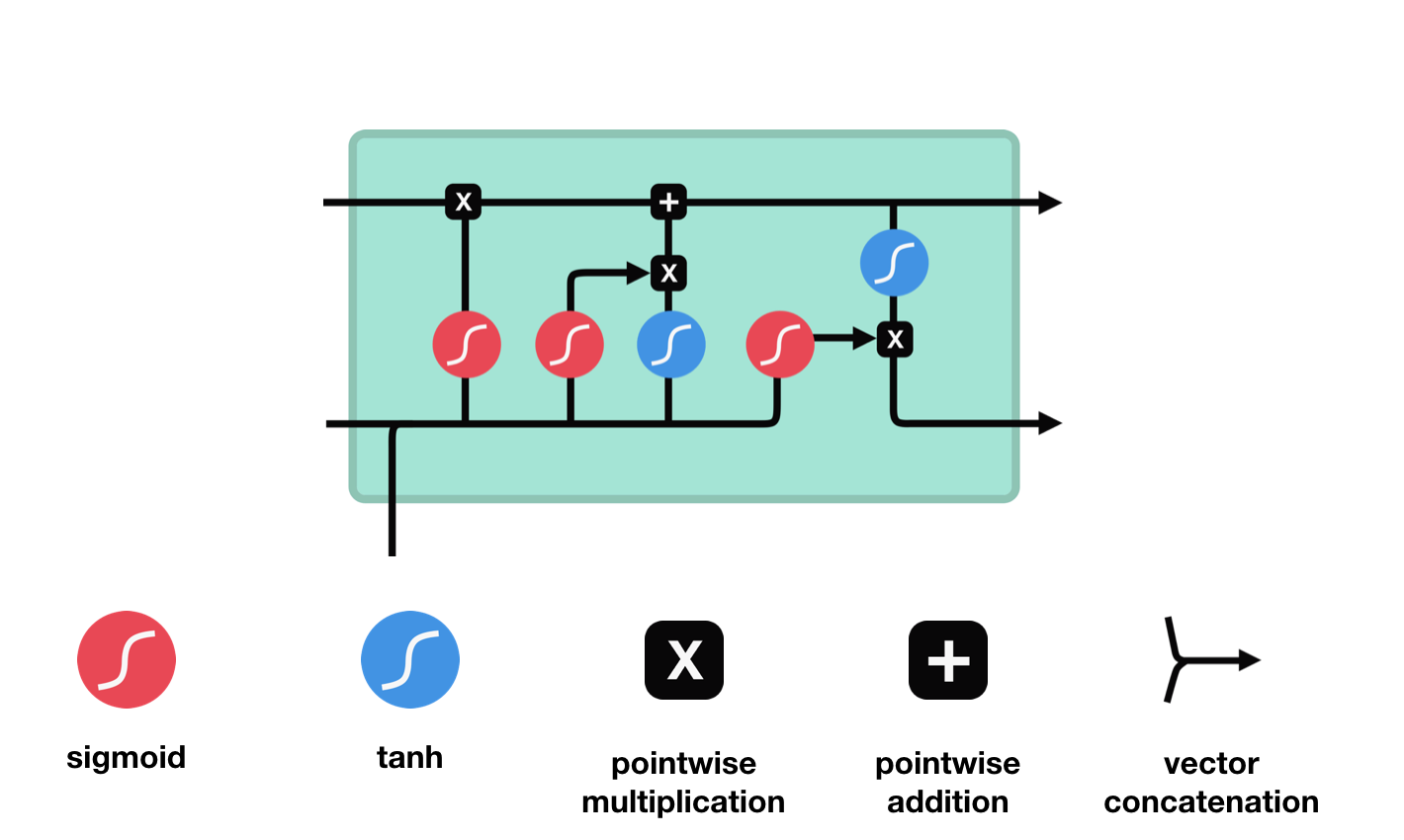LSTM Cell and It’s Operations

These operations are used to allow the LSTM to keep or forget information. Now looking at these operations can get a little overwhelming so we’ll go over this step by step.

## Core Concept

The core concept of LSTM’s are the cell state, and it’s various gates. The cell state act as a transport highway that transfers relative information all the way down the sequence chain. You can think of it as the “memory” of the network. The cell state, in theory, can carry relevant information throughout the processing of the sequence. So even information from the earlier time steps can make it’s way to later time steps, reducing the effects of short-term memory. As the cell state goes on its journey, information get’s added or removed to the cell state via gates. The gates are different neural networks that decide which information is allowed on the cell state. The gates can learn what information is relevant to keep or forget during training.

## Sigmoid

Gates contains sigmoid activations. A sigmoid activation is similar to the tanh activation. Instead of squishing values between -1 and 1, it squishes values between 0 and 1. That is helpful to update or forget data because any number getting multiplied by 0 is 0, causing values to disappears or be “forgotten.” Any number multiplied by 1 is the same value therefore that value stay’s the same or is “kept.” The network can learn which data is not important therefore can be forgotten or which data is important to keep.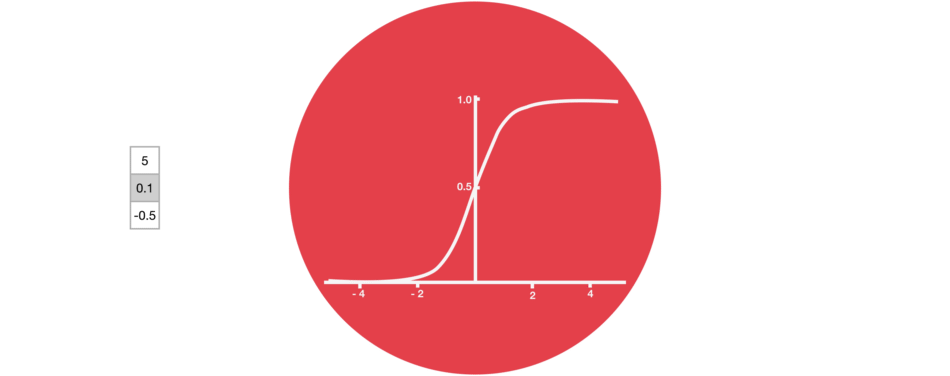Sigmoid squishes values to be between 0 and 1

Let’s dig a little deeper into what the various gates are doing, shall we? So we have three different gates that regulate information flow in an LSTM cell. A forget gate, input gate, and output gate.

## Forget gate

First, we have the forget gate. This gate decides what information should be thrown away or kept. Information from the previous hidden state and information from the current input is passed through the sigmoid function. Values come out between 0 and 1. The closer to 0 means to forget, and the closer to 1 means to keep.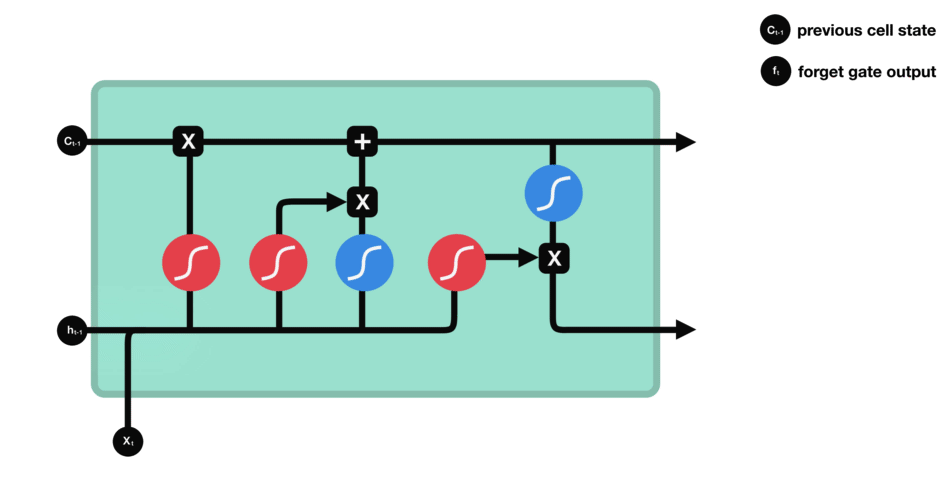Forget gate operations## Input Gate

To update the cell state, we have the input gate. First, we pass the previous hidden state and current input into a sigmoid function. That decides which values will be updated by transforming the values to be between 0 and 1. 0 means not important, and 1 means important. You also pass the hidden state and current input into the tanh function to squish values between -1 and 1 to help regulate the network. Then you multiply the tanh output with the sigmoid output. The sigmoid output will decide which information is important to keep from the tanh output.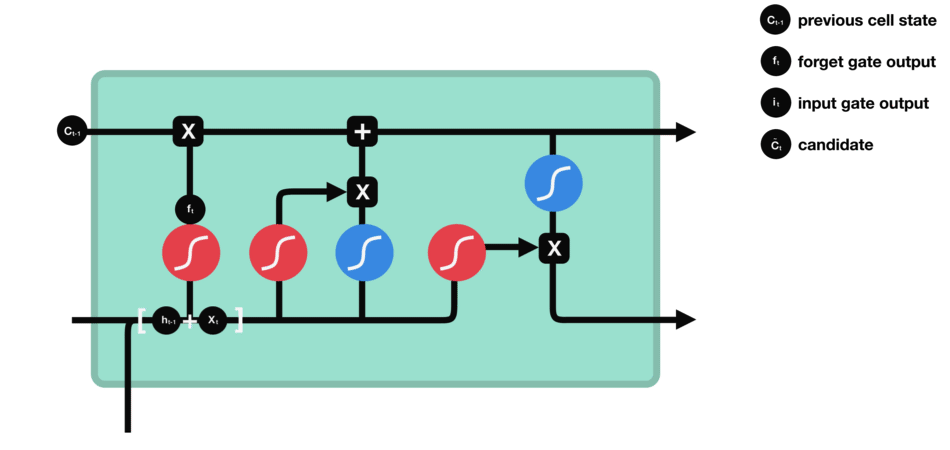Input gate operations## Cell State

Now we should have enough information to calculate the cell state. First, the cell state gets pointwise multiplied by the forget vector. This has a possibility of dropping values in the cell state if it gets multiplied by values near 0. Then we take the output from the input gate and do a pointwise addition which updates the cell state to new values that the neural network finds relevant. That gives us our new cell state.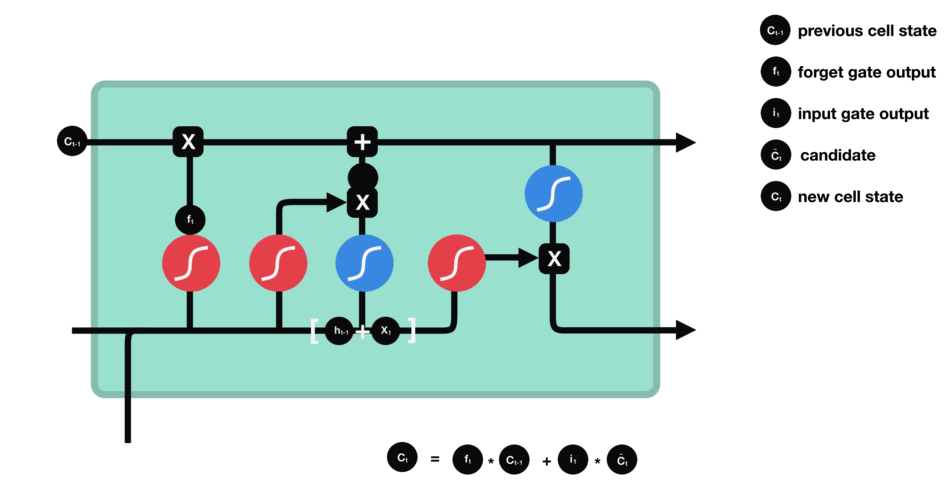Calculating cell state## Output Gate

Last we have the output gate. The output gate decides what the next hidden state should be. Remember that the hidden state contains information on previous inputs. The hidden state is also used for predictions. First, we pass the previous hidden state and the current input into a sigmoid function. Then we pass the newly modified cell state to the tanh function. We multiply the tanh output with the sigmoid output to decide what information the hidden state should carry. The output is the hidden state. The new cell state and the new hidden is then carried over to the next time step.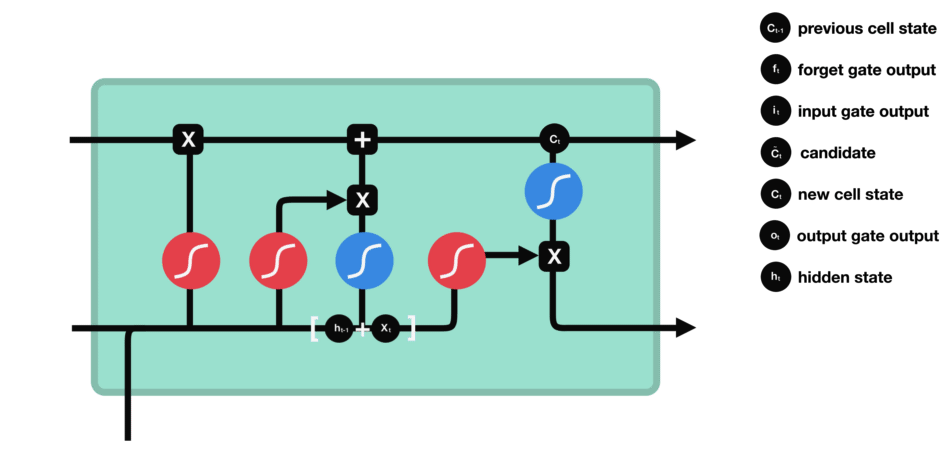output gate operationsIn summary, the Forget gate decides what is relevant to keep from prior steps. The input gate decides what information is relevant to add from the current step. The output gate determines what the next hidden state should be.

## Code Demo

For those of you who understand better through seeing the code, here is an example using python pseudo code.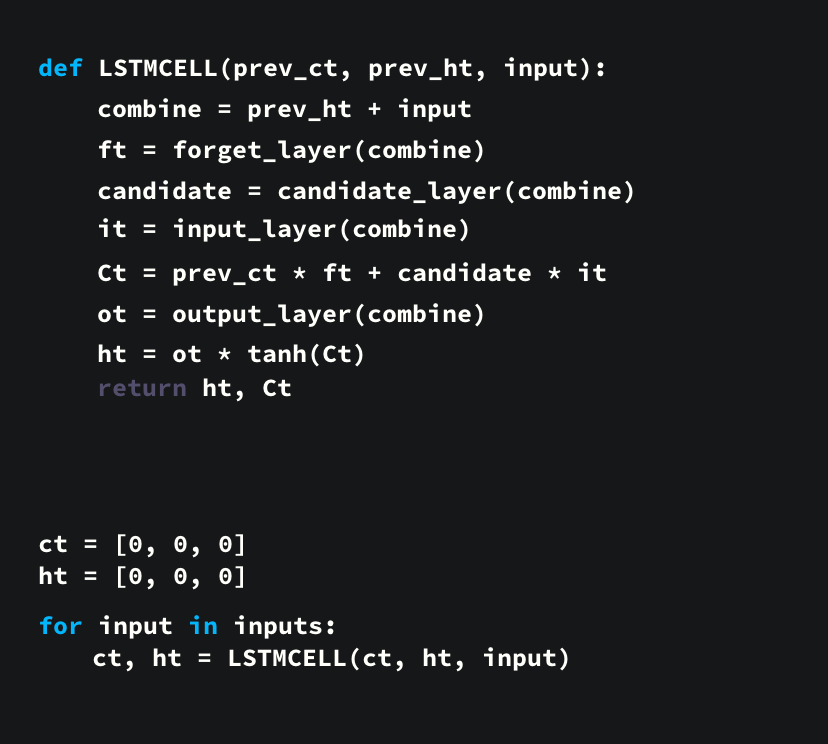python pseudo code